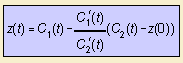# roulette

## mainGiven two curves C1 and C2 and a point P attached to curve C2. Now let curve C2 roll along curve C1, without slipping. Then P describes a roulette 1).
When P is on curve C2, the curve is called a point-roulette. When P is on a line attached to C2, the curve is called a line-roulette.

When working in the complex plane and both curves C1 and C2 are expressed as function of the arc length, the resulting curve z can be written as a function of C1 and C2 2).

The first to describe these curves where Besant (1869) and Bernat (1869), respectively.
The following roulettes can be distinguished, for a curve C2 that is rolling over a curve C1:

 fixed curve C1 rolling curve C2 fixed point on curve C2 roulette any curve C line on line involute of curve C catenary line center of catenary line circle circle (on outside) on circle epicycloid circle (on inside) on circle hypocycloid line circle on circle cycloid circle outside circle prolate cycloid circle inside circle curtate cycloid cycloid center ellipse ellipse focus elliptic catenary hyperbola focus hyperbolic catenary hyperbolic spiral pole tractrix involute of a circle center parabola involute of a circle any point circle logarithmic spiral any point line parabola focus catenary parabola (equal) parabola vertex cissoid

The point-roulettes for which a circle rolls on a line or on another circle, are known as cycloidal curves.

Some authors name the roulette a spirographic curve.

The same curves can be defined as a glissette 3): as the locus of a point or a envelope of a line which slides between two given curves C1 and C2.
An wide-known example of a glissette is the astroid.

notes

1) rouler (Fr.) = to roll.
In Dutch: rolkromme.

2) The formula follows from the isometric insight that C1(t) - z(t) / C2(t) - z(0) = C1'(t) / C2'(t).

Example for the cycloid: C1(t) = t and C2(t) = i - i e it where t is the arc length.

3) glisser (Fr.) = to glide.